Question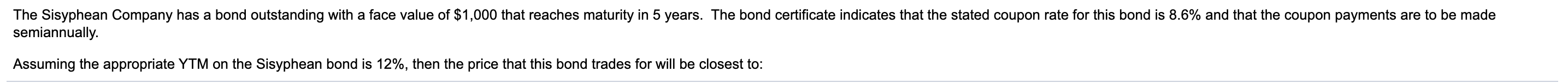The price of the bond is computed as shown below:

The coupon payment is computed as follows:

= Coupon rate / 2 x \$ 1,000 (Since the payments are semi annual hence divided by 2)

= 8.6% / 2 x \$ 1,000

= \$ 43

The YTM is computed as follows:

= 12% / 2 (Since the payments are semi annual hence divided by 2)

= 6% or 0.06

The n is computed as follows:

= 5 x 2 (Since the payments are semi annual hence multiplied by 2)

= 10

So, the price of the bond will be as follows:

= \$ 43 / 1.061 + \$ 43 / 1.062 + \$ 43 / 1.063 + \$ 43 / 1.064 + \$ 43 / 1.065 + \$ 43 / 1.066 + \$ 43 / 1.067 + \$ 43 / 1.068 + \$ 43 / 1.069 + \$ 43 / 1.0610 + \$ 1,000 / 1.0610

= \$ 874.88 Approximately

Feel free to ask in case of any query relating to this question

#### Earn Coins

Coins can be redeemed for fabulous gifts.

Similar Homework Help Questions
• ### The Sisyphean Company has a bond outstanding with a face value of \$1,000 that reaches maturity...

The Sisyphean Company has a bond outstanding with a face value of \$1,000 that reaches maturity in 8 years. The bond certificate indicates that the stated coupon rate for this bond is 8.6​% and that the coupon payments are to be made semiannually. Assuming that this bond trades for \$ 963​, then the YTM for this bond is closest​ to:

• ### The Sisyphean Company has a bond outstanding with a face value of \$1,000 that reaches maturity...

The Sisyphean Company has a bond outstanding with a face value of \$1,000 that reaches maturity in 9 years. The bond certificate indicates that the stated coupon rate for this bond is 8.8​% and that the coupon payments are to be made semiannually. Assuming the appropriate YTM on the Sisyphean bond is 10.3​%, then the price that this bond trades for will be closest​ to:

• ### The Sisyphean Company has a bond outstanding with a face value of \$1,000 that reaches maturity...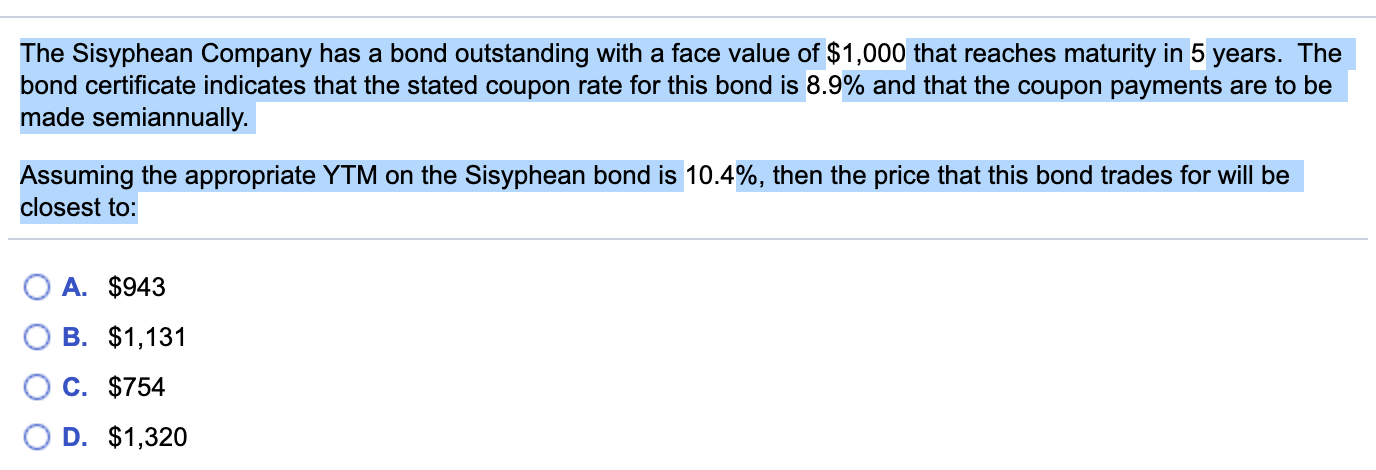The Sisyphean Company has a bond outstanding with a face value of \$1,000 that reaches maturity in 5 years. The bond certificate indicates that the stated coupon rate for this bond is 8.9% and that the coupon payments are to be made semiannually. Assuming the appropriate YTM on the Sisyphean bond is 10.4%, then the price that this bond trades for will be closest to: O A. \$943 OB. \$1,131 O c. \$754 OD. \$1,320

• ### The Sisyphean Company has a bond outstanding with a face value of \$1,000 that reaches maturity...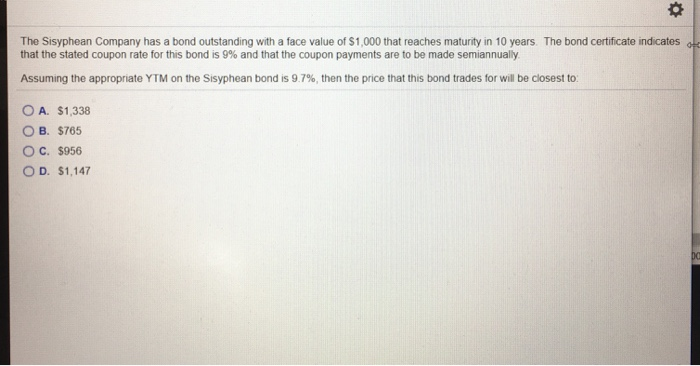The Sisyphean Company has a bond outstanding with a face value of \$1,000 that reaches maturity in 10 years. The bond certificate indicates old that the stated coupon rate for this bond is 9% and that the coupon payments are to be made semiannually Assuming the appropriate YTM on the Sisyphean bond is 9.7%, then the price that this bond trades for will be closest to O A. \$1,338 OB. \$765 O C. \$956 OD. \$1,147

• ### The Sisyphean Company has a bond outstanding with a face value of \$1,000 that reaches maturity in 15 years. The bond cer...

The Sisyphean Company has a bond outstanding with a face value of \$1,000 that reaches maturity in 15 years. The bond certificate indicates that the stated coupon rate for this bond is 8.8% and that the coupon payments are to be made semiannually. Assuming the appropriate YTM on the Sisyphean bond is 10.8% , then the price that this bond trades for will be closest to: a. \$853 b. \$1,194 c. \$682 d. \$1,024

• ### The Sisyphean Company has a bond outstanding with a face value of \$ 1000 that reaches...

The Sisyphean Company has a bond outstanding with a face value of \$ 1000 that reaches maturity in 8 years. The bond certificate indicates that the stated coupon rate for this bond is 9​% and that the coupon payments are to be made semiannually. Assuming the appropriate YTM on the Sisyphean bond is 10.3​%, then the price that this bond trades for will be closest​ to:

• ### The Sisyphean Company has a bond outstanding with a face value of \$5,000 that reaches maturity...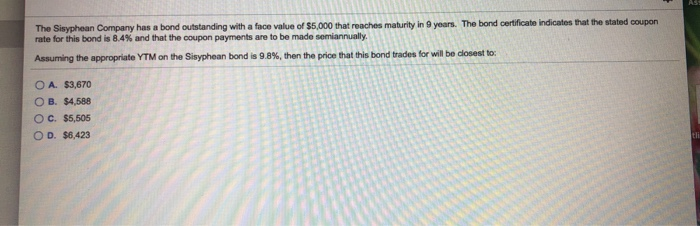The Sisyphean Company has a bond outstanding with a face value of \$5,000 that reaches maturity in 9 years. The bond certificate indicates that the stated coupon rate for this bond is 84% and that the coupon payments are to be made semiannually Assuming the appropriate YTM on the Sisyphean bond is 9.8%, then the price that this bond trades for will be closest to O A. \$3,670 O B. \$4,588 OC. \$5,505 OD. \$6,423

• ### The Sisyphean Company has a bond outstanding with a face value of \$5,000 that reaches maturity...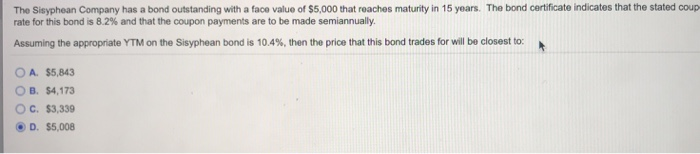The Sisyphean Company has a bond outstanding with a face value of \$5,000 that reaches maturity in 15 years. The bond certificate indicates that the stated coup rate for this bond is 8.2% and that the coupon payments are to be made semiannually. Assuming the appropriate YTM on the Sisyphean bond is 10.4%, then the price that this bond trades for will be closest to: O A. \$5,843 OB. \$4,173 OC. \$3,339 D. \$5,008

• ### The Sisyphean Company has a bond outstanding with a face value of \$5,000 that reaches maturity in 15 years. The bond cer...

The Sisyphean Company has a bond outstanding with a face value of \$5,000 that reaches maturity in 15 years. The bond certificate indicates that the stated coupon rate for this bond is 88​% and that the coupon payments are to be made semiannually. Assuming the appropriate YTM on the Sisyphean bond is 11.5​%, then the price that this bond trades for will be closest​ to: A.\$4,515 B.\$3,010 C.\$5,268 D. \$ 3763

• ### The Sisyphean Company has a bond outstanding with a face value of \$ 5,000 that reaches...

The Sisyphean Company has a bond outstanding with a face value of \$ 5,000 that reaches maturity in 10 years. The bond certificate indicates that the stated coupon rate for this bond is 9.8​% and that the coupon payments are to be made semiannually. Assuming that this bond trades for \$ 5,198​, then the YTM for this bond is closest​ to: